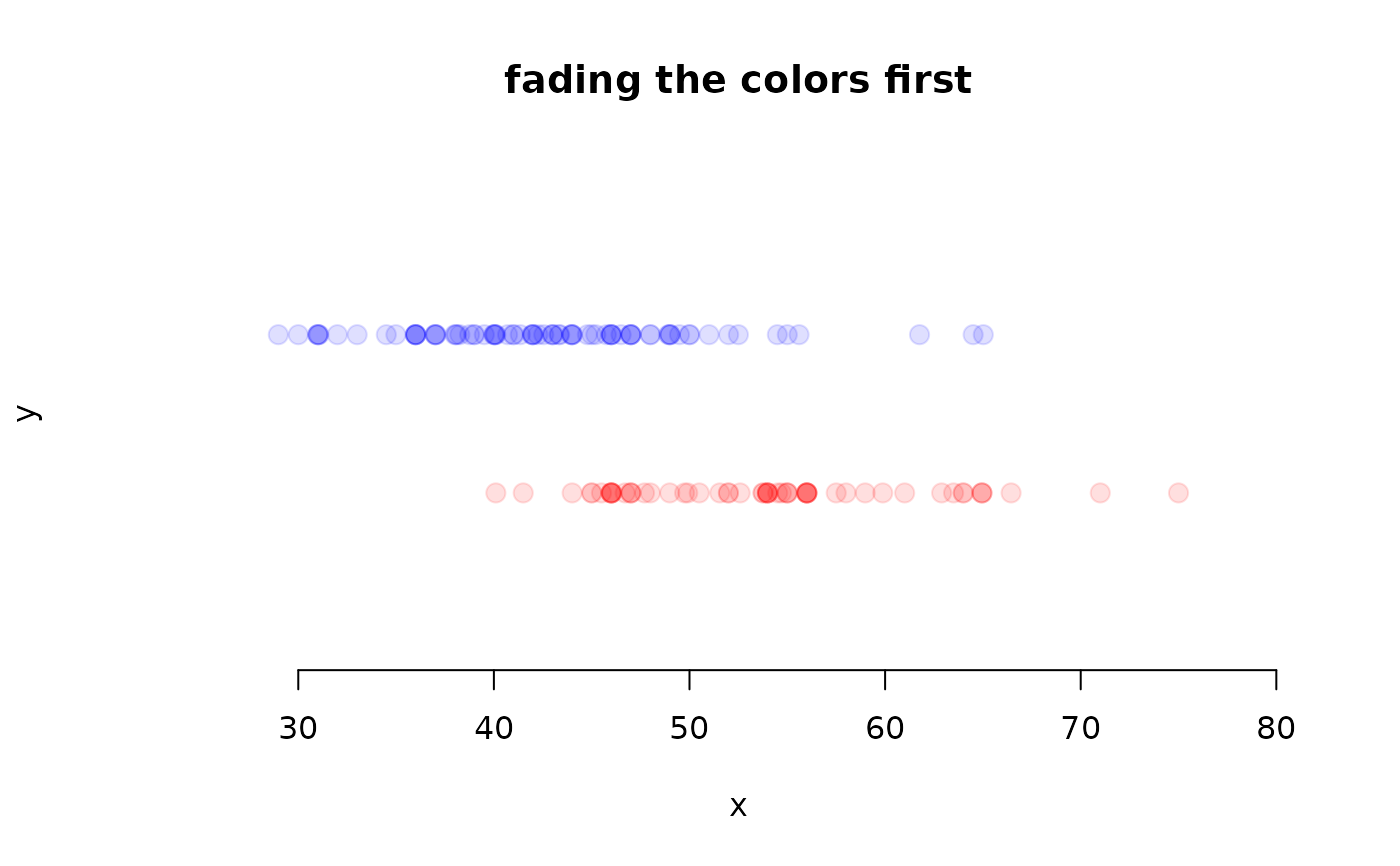Fade colors so they are transparent.

## Usage

fadeColor(col, fade = "FF")

## Arguments

col

An integer, color name, or RGB hexadecimal.

The amount to fade col. This value should be a character in hexadecimal from '00' to 'FF'. The smaller the value, the greater the fading.

David Diez

## Examples


data(mariokart)
new <- mariokart$cond == "new" used <- mariokart$cond == "used"

# ===> color numbers <===#
dotPlot(mariokart$total_pr[new], ylim = c(0, 3), xlim = c(25, 80), pch = 20, col = 2, cex = 2, main = "using regular colors" ) dotPlot(mariokart$total_pr[used], at = 2, add = TRUE, col = 4, pch = 20, cex = 2)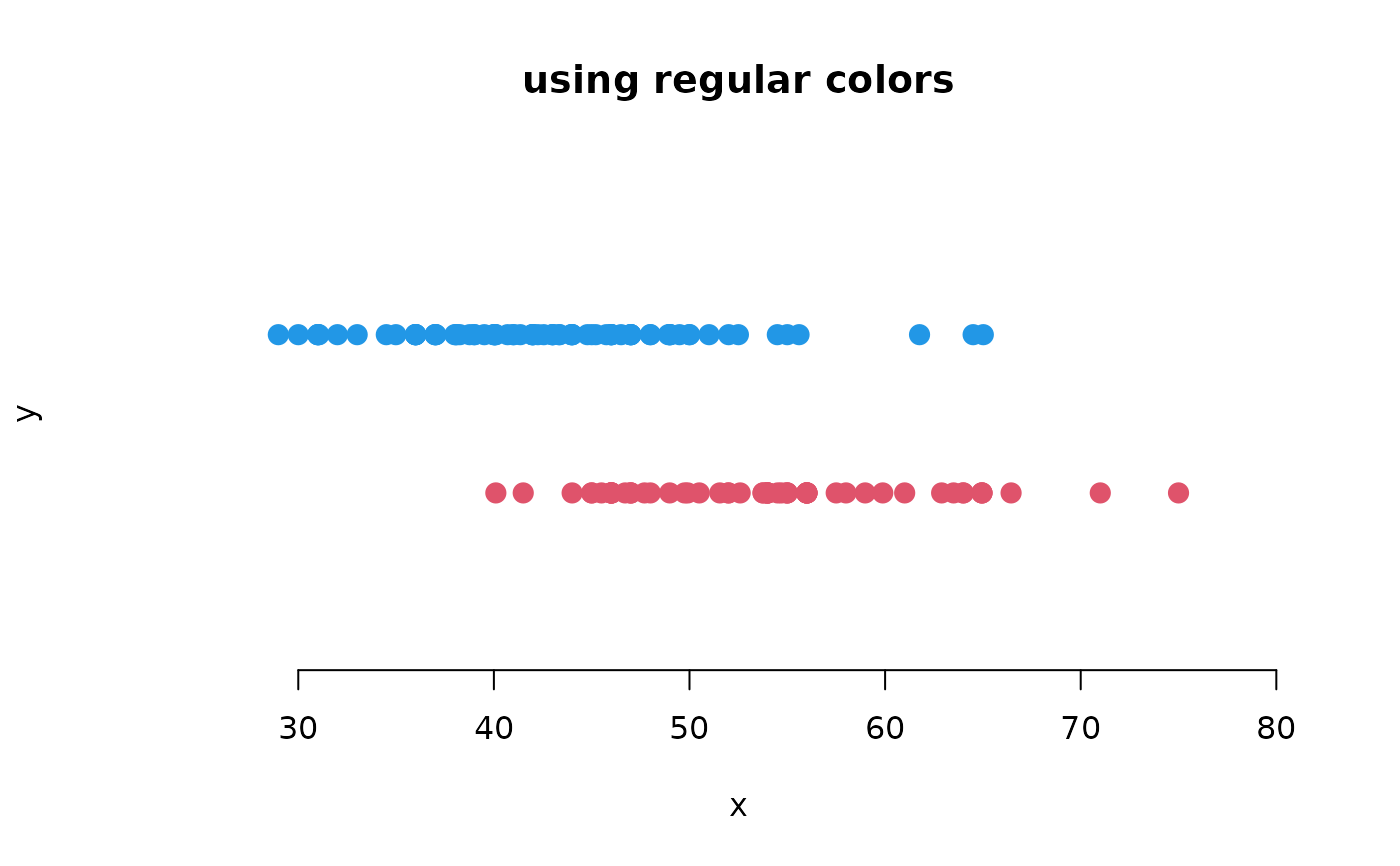dotPlot(mariokart$total_pr[new], ylim = c(0, 3), xlim = c(25, 80), col = fadeColor(2, "22"), pch = 20, cex = 2, main = "fading the colors first" ) dotPlot(mariokart$total_pr[used],
at = 2, add = TRUE,
col = fadeColor(4, "22"), pch = 20, cex = 2
)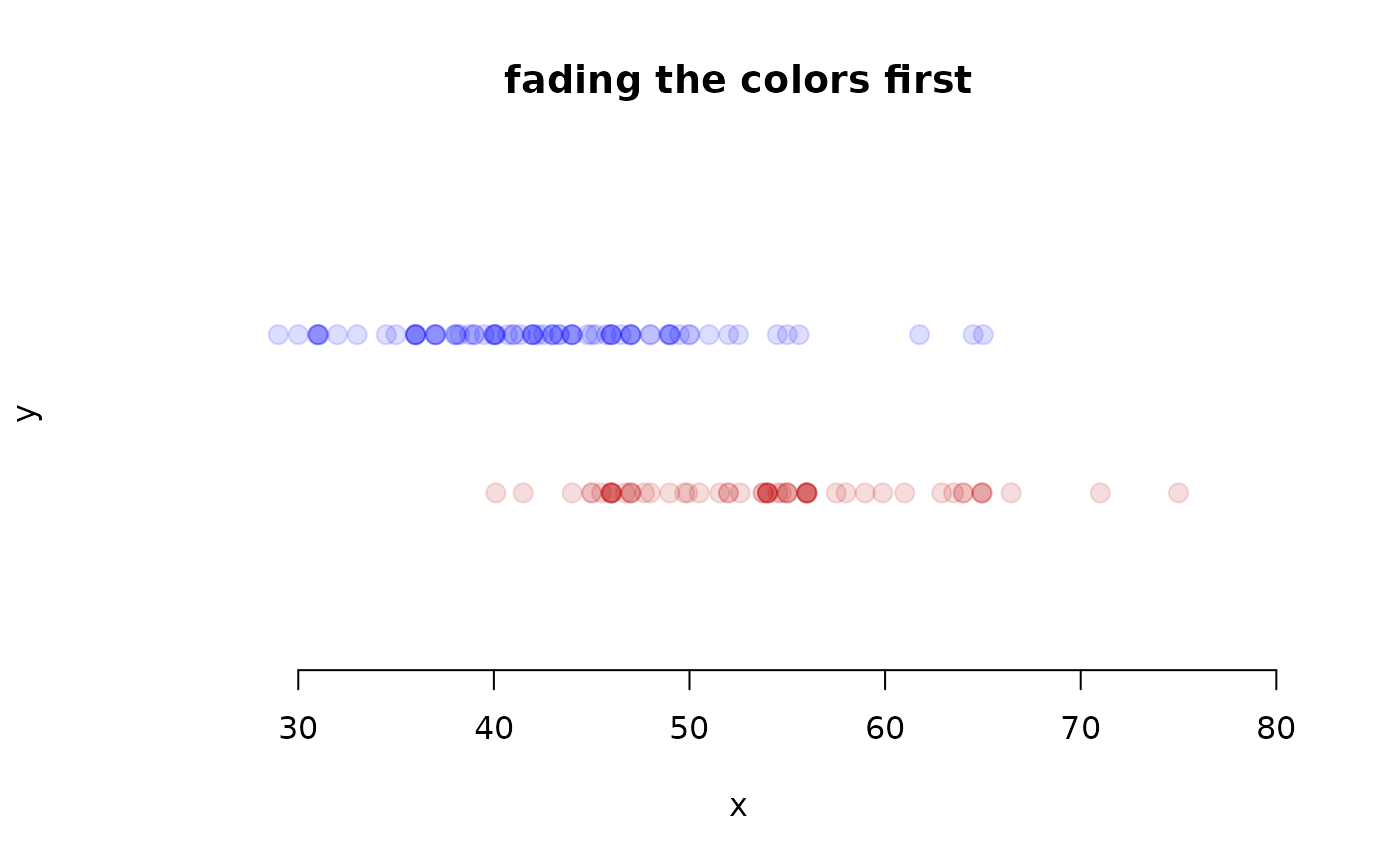# ===> color names <===#
dotPlot(mariokart$total_pr[new], ylim = c(0, 3), xlim = c(25, 80), pch = 20, col = "red", cex = 2, main = "using regular colors" ) dotPlot(mariokart$total_pr[used], at = 2, add = TRUE, col = "blue", pch = 20, cex = 2)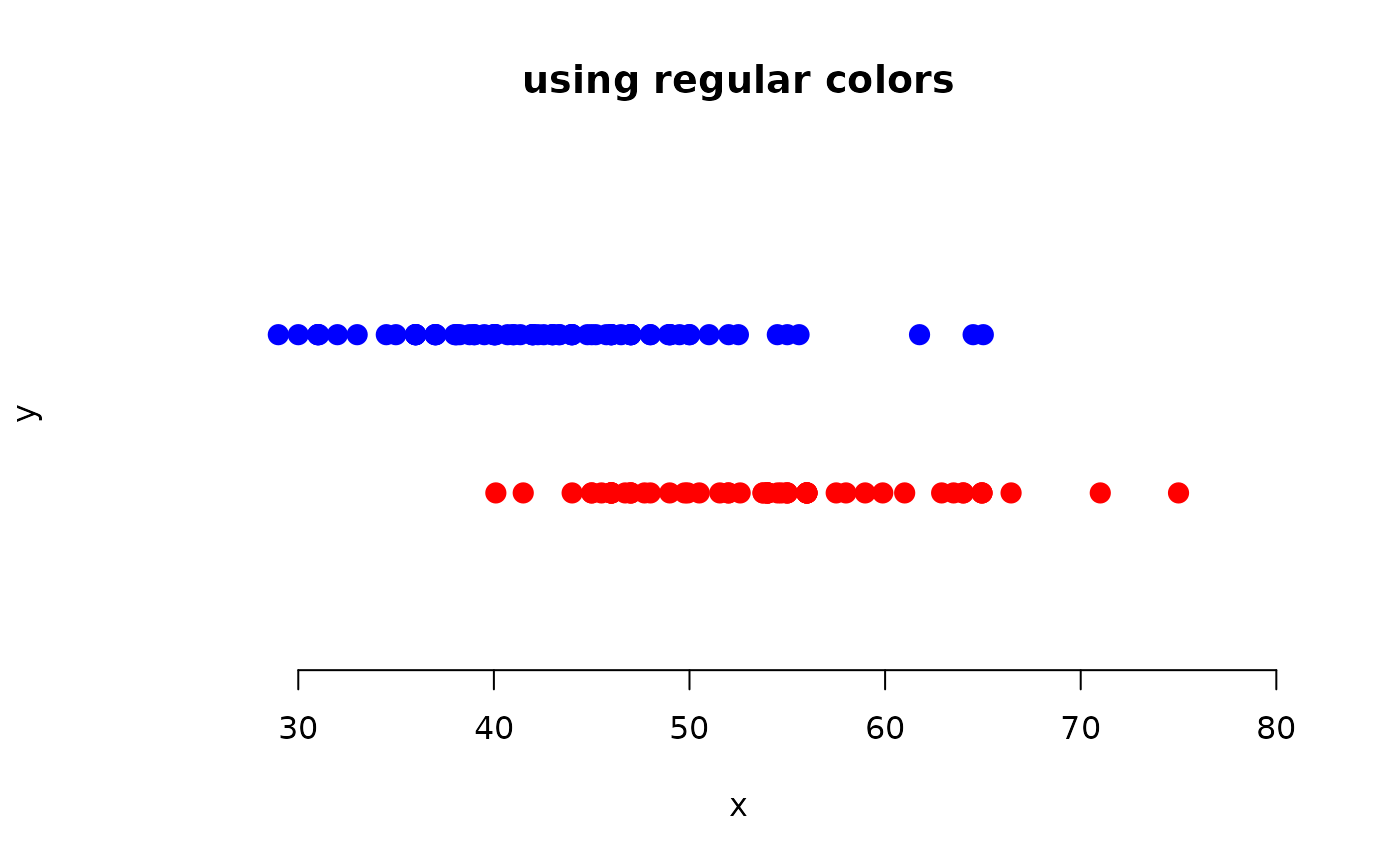dotPlot(mariokart$total_pr[new], ylim = c(0, 3), xlim = c(25, 80), col = fadeColor("red", "22"), pch = 20, cex = 2, main = "fading the colors first" ) dotPlot(mariokart$total_pr[used],
at = 2, add = TRUE,
col = fadeColor("blue", "22"), pch = 20, cex = 2
)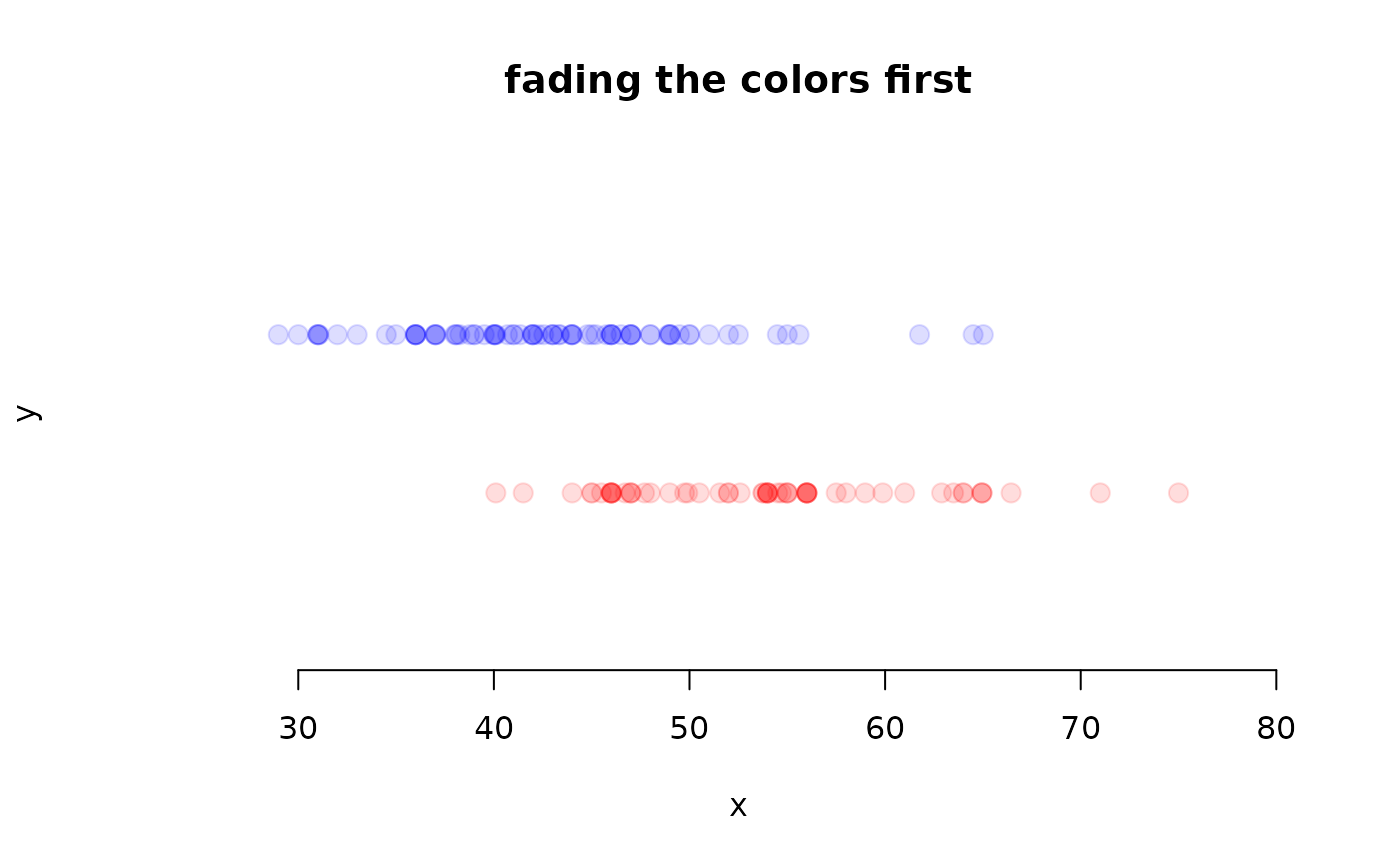dotPlot(mariokart$total_pr[new], ylim = c(0, 3), xlim = c(25, 80), pch = 20, col = "#FF0000", cex = 2, main = "using regular colors" ) dotPlot(mariokart$total_pr[used],
at = 2, add = TRUE, col = "#0000FF", pch = 20,
cex = 2
)dotPlot(mariokart$total_pr[new], ylim = c(0, 3), xlim = c(25, 80), col = fadeColor("#FF0000", "22"), pch = 20, cex = 2, main = "fading the colors first" ) dotPlot(mariokart$total_pr[used],
at = 2, add = TRUE,
col = fadeColor("#0000FF", "22"), pch = 20, cex = 2
)# ===> alternative: rgb function <===#
dotPlot(mariokart$total_pr[new], ylim = c(0, 3), xlim = c(25, 80), pch = 20, col = rgb(1, 0, 0), cex = 2, main = "using regular colors" ) dotPlot(mariokart$total_pr[used],
at = 2, add = TRUE, col = rgb(0, 0, 1),
pch = 20, cex = 2
)dotPlot(mariokart$total_pr[new], ylim = c(0, 3), xlim = c(25, 80), col = rgb(1, 0, 0, 1 / 8), pch = 20, cex = 2, main = "fading the colors first" ) dotPlot(mariokart$total_pr[used],
at = 2, add = TRUE,
col = rgb(0, 0, 1, 1 / 8), pch = 20, cex = 2
)